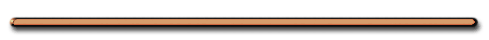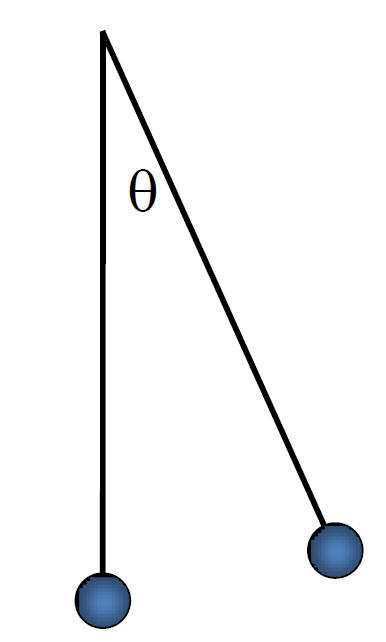HomePeriod of a Pendulum   - determined by  ....     LengthT = 1/fFinding the height of a pendulum from a pendulum angleKE1 + PE1 = KE2 + PE2   Ex) A pendulum is pulled back at an angle of 10 degrees from the vertical and is released. When the ball reaches the lowest point, it has a velocity of 2 m/s.   What is the frequency of the pendulum?   KE1 + PE1 = KE2 + PE2   mgh = = 1/2mv2   mg(L - Lcosq) = 1/2mv2       L = 13.2 mT = 7.2 sec   T = 1/f     T = .14 Hz     Giancoli p. 317) 2, 3, 5, 8, 28, 30, 32©Tony Mangiacapre., - All Rights Reserved [Home] Established 1995 Use any material on this site (w/ attribution)